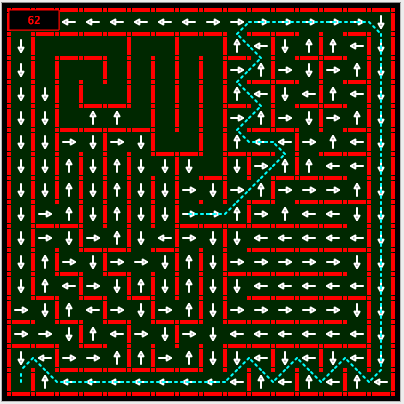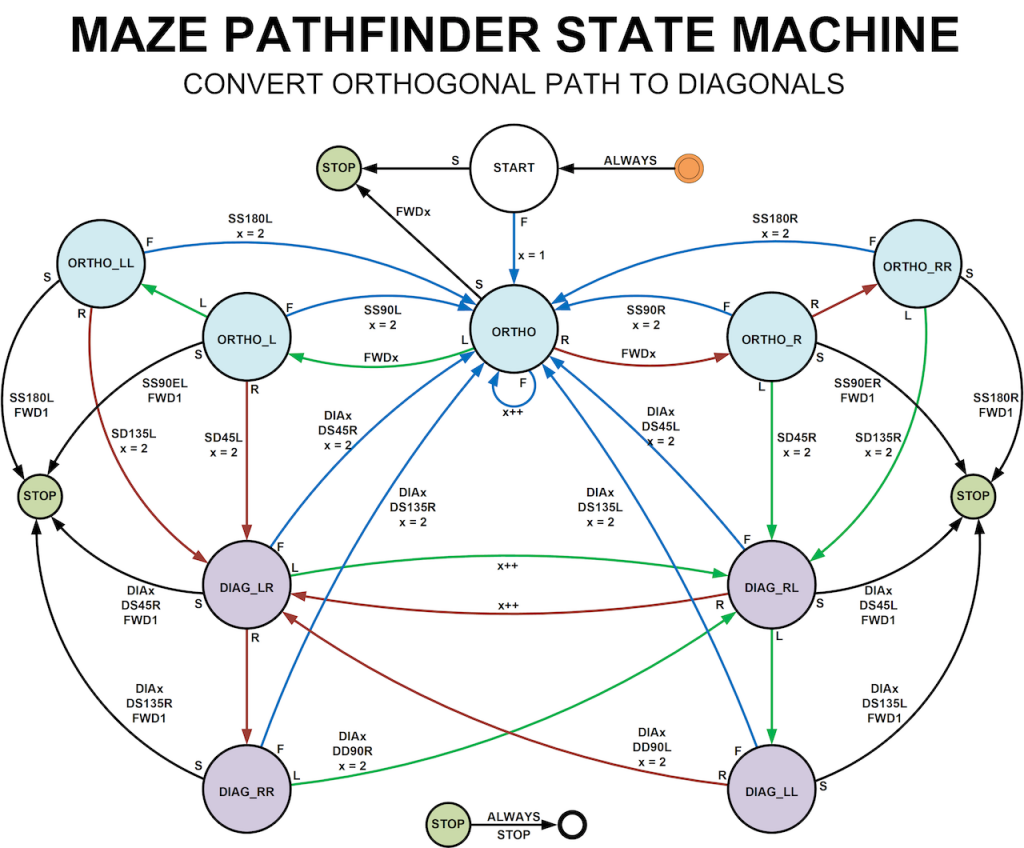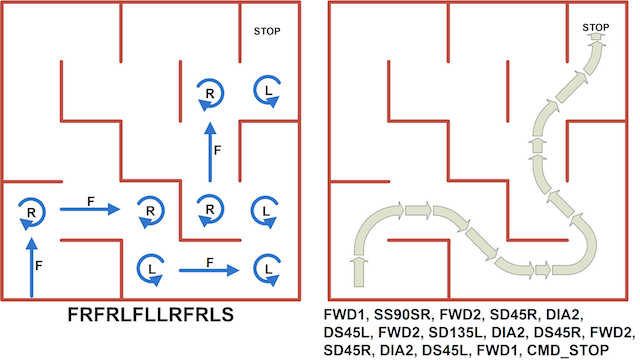To get the shortest possible run times, a micromouse must be able to make full use of diagonal paths. By running a diagonal, slow, tricky turns are avoided and the distance is much less. No competitive micromouse can ignore diagonals.

## Diagonal Paths

From the point of view of a human observer, it is pretty obvious what the diagonal path must be. From the perspective of the micromouse, it is far from clear. After solving the maze, the micromouse will have a map that has, for each cell, a cost and or a direction. The cost may be a simple distance from the goal or it may be a number that represents how long it will take to get to the goal from that cell. If directions are stored, they will tell the micromouse which way it should be heading in that cell to be on the lowest cost path to the goal. This example shows a diagonal path calculated from the goal back to the start in the All Japan 2007 expert final maze.The method used here assumes that the micromouse has used the cost or direction data to generate a simple path consisting of one of only four instructions:

F – move forward one cell
R – turn right 90 degrees in place then go forward one cell
L – turn left 90 degrees in place then go forward one cell
S – stop moving

So a simple path might be stored as a string like this “FFRFFFLRFLFFS”. This is described in more detail in a previous post about finding a smooth path to the goal. the path described in that post is an orthogonal path. that is, it consists only of straights that run North-South and East-West with smooth, 90 degree or 180 degree turns.

That post describes how a smooth path can be generated by using a state machine to work through a simple instruction list, building a list of commands for the mouse to execute. This approach is particularly convenient. Not only is it flexible in how the paths can be generated, it makes testing a micromouse much easier. The control software can be developed step by step. Start with simple commands to move forwards one cell and turn in place. Add the ability to move forward a fixed distance. Add simple smooth turns. Combine these into complex paths. Add diagonal turns. Before you know it, you have a fast (hopefully) and flexible micromouse with modular, easily tested and maintained code.

If you have not read the post on generating smooth turns, read it now as this article builds on that and uses it as a starting point.

If you are not comfortable with state machines, read the introduction to state machines first.

## Extra commands for running diagonal paths

Once you are happy about how smooth paths can be generated using a state machine, the task of generating diagonal paths becomes much easier. In the smooth turn state machine, there are a number of choices missing. Some of these are errors and some lead to diagonal path solutions. For example, if the input list contains the string “FLLL”, that would represent three consecutive left turns. Remember that each turn is in-place has an implied straight after it. In a real maze, that must be an error except in one specific location. The rules state that each post must have a wall attached. Three consecutive turns would require that there are no walls around a post and that can only happen in the centre of the maze. A contest run does not need to go right around the post so we can safely treat “FLLL” and “FRRR” as errors. Notice that it is not possible to tell that this is an error until the third turn has been seen since “FRRF” would be a 180 degree smooth turn.

However, what about “FLLR”? That is perfectly legal and represents a 135 degree left turn from straight to diagonal. Similarly, “FRRL” is a 135 degree right turn from straight to diagonal. In the post about smooth paths, I listed some command names that I use to describe turns. Here is a more complete list:

• IP90R In-place 90 degree turn
• IP90L
• IP180R In-place 180 degree turn
• IP180L
• SS90SR straight-to-straight 90 degree smooth turn
• SS90SL
• SS180R straight-to-straight 180 degree smooth turn
• SS180L
• SD45R straight-to-diagonal 45 degree turn
• SD45L
• SD135R straight-to-diagonal 135 degree turn
• SD135L
• DS45R diagonal-to-straight 45 degree turn
• DS45L
• DS135R diagonal-to-straight 135 degree turn
• DS135L
• DD90R diagonal-to-diagonal 90 degree turn
• DD90L
• SS90ER straight-to-straight 90 degree explore turn (sharper than smooth turn)
• SS90EL

Names are very descriptive to make it easier to see what is going on. The mouse has code that will print out a list of commands as test using these names. A function can be given an array with commands using these mnemonics for execution. All this makes testing specific paths very easy and allows me to visualise the path generated by the pathfinding code. Writing tests while developing the diagonal pathfinder is also made much easier.

## The complete state machine

Once the required turn types have been identified, it is not too hard to see what sequences of input instructions will generate the outputs for diagonal paths. All that remains then is to extend the smooth path state machine to allow it to generate fully diagonal paths.This is a much more complex state diagram but it should be easy enough to follow if taken step-by-step. If you compare it with the state machine in the smooth pathfinding article (here) you will see that it is just an extension of that one. The states have been given names that should help to see what is happening. For example, a sequence of “LRLRLR” instructions indicate a diagonal path in the output. There are two states, DIAG_LR anf DIAG_RL, that show that the length of the diagonal path is simply extended by one cell as each successive turn is found. Whenever the sequence “LRRL” is found, it represents a DD90R turn. Normally, that would be found while on a diagonal so the machine is either in state DIAG_LR or DIAG_RL. It can also occur immediately after a turn into a diagonal so it can also be detected from ORTHO_L and ORTHO_LL. try it out for yourself. the states with names beginning ORTHO_ show the mouse running along a North-South or East-West straight. Those states with names beginning DIAG_ show the mouse running a diagonal straight.

## A diagonal path example

Here is a small but complete example of a path generated by the state machineSample source code and test data can be found on github:

https://github.com/micromouseonline/diagonal-pathfinder

This site uses Akismet to reduce spam. Learn how your comment data is processed.